# Polarizers

Excerpt from Field Guide to Polarization

The polarizing element that changes the amplitude is a linear polarizer, which is characterized by two absorption coefficients that differ along the x- and y-axes, respectively. The absorption coefficients in the amplitude domain are defined by px and py and are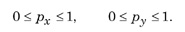The value of 0 represents total absorption (no transmission) and the value of 1 represents total transmission (no absorption).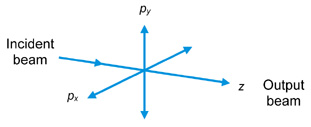The Mueller matrix for a linear polarizer (the polarization matrix form used in the intensity domain) is found to be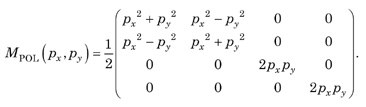For an ideal linear polarizer there is complete transmission along one axis and no transmission along the orthogonal axis. The Mueller matrix for an ideal linear polarizer with its transmission axis along the x-axis is px = 1 and py = 0. Therefore,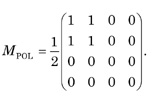For an ideal linear polarizer in which the transmission axis is along the y-axis, the Mueller matrix is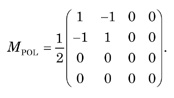The following figure shows a pair of crossed polarizers (The transmission axes are orthogonal to each other.)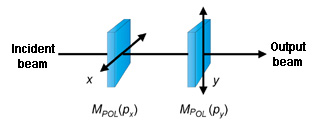The Mueller matrix for the pair is M = MPOL(py)• MPOL(px)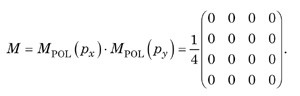This is a null matrix and shows that no light emerges when the linear polarizers are crossed. Another interesting application occurs for equal absorption along both axes [a neutral density (ND) filter]. In this case, px = py = p, therefore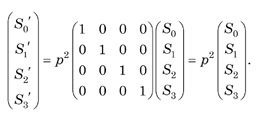The polarization state of the incident beam remains unchanged, but the intensity is reduced by a factor of p2.

Citation:

E. Collett, Field Guide to Polarization, SPIE Press, Bellingham, WA (2005).